# Number Systems NCERT Solutions : Class 9 Maths

## NCERT Solutions for Class 9 Maths : Number SystemsFilter
Filters :
Classes
•
•
•
•
•
•
•
Chapters
•
•
•
•
•
•
•
•
•
•
• 7 More
Exercises
•
•
•
•
•
•
•

Doubtnut one of the best online education platform provides free NCERT Solutions of Maths for Class 9 Number Systems which are solved by our maths experts as per the NCERT (CBSE) guidelines. We provide the solutions as video solutions in which concepts are also explained along with answer which will help students while learning , preparing for exams and in doing homework. These Solutions will help to revise complete syllabus and score more marks in examinations.

Get here free, accurate and comprehensive NCERT Solutions for Class 9 Maths Number Systems which have been reviewed by our maths counsellors as per the latest edition following up the CBSE guidelines. We provide video solutions in which solutions to all the questions of NCERT Class 9 Maths textbook are explained in step by step and detailed way.

## The Topics of the Chapter Number Systems are :

EXAMPLES BASED ON LAWS OF EXPONENTS

INTEGRAL EXPONENTS

LAWS OF INTEGRAL EXPONENTS

CONVERSION OF DECIMAL NO. INTO RATIONAL NO. OF THE FORM M/N

IRRATIONAL NUMBERS

REPRESENTING IRRATIONAL NUMBER ON THE NUMBER LINE.

DECIMAL REPRESENTATION OF RATIONAL NUMBER

REVISION EXAMPLE OF PREVIOUS CLASS

INTRODUCTION

RATIONALISATION OF DENOMINATOR

LAWS OF RATIONAL EXPONENTS

BRIEF REVIEW OF NUMBERS

SOME IDENTITIES OF RATIONAL NUMBERS

SOME USEFUL RESULTS ON IRRATIONAL NUMBERS

EXISTENCE OF A SQUARE ROOT OF A POSITIVE REAL NUMBER

VISUALISATION OF REPRESENTATION OF REAL NO. BY SUCCESSIVE MAGNIFICATION

TYPES OF EXAMPLES

It contains these exercises along with solved examples. All the exercises are solved in the video. Select the exercise to view the solutions exercisewise of the Chapter Number Systems:

## NCERT Solutions Class 9 Maths Chapter Number Systems Exercises:

We have covered all the exercises and also Solved examples in the videos. Along with the practise exercise Students should also practise solved examples to clear the concepts of the Number Systems. If incase you have any doubt you can watch the solutions for the given questions.Watch the solutions for the given questions in which it is also explained in the video steps to solve the questions along with answers.

# NCERT CLASS 9 SOLUTIONS CHAPTER 1 NUMBER SYSTEM

Students must have attained complete knowledge about natural numbers and whole numbers in prior classes. In Class 9 Chapter 1, Number System, the students will be made familiar with the rational numbers, irrational numbers, and number line. This chapter of the number system focuses on all these different aspects in depth and several other topics. The NCERT book offers the students with perfect and accurate information and due to this reason; it is one of the most favorite books of students.

Though there are a number of different writers’ books that are available in the market, but for the CBSE students, it is paramount that they give the NCERT books the maximum priority, as it is covers the entire syllabus prescribed by CBSE board. The NCERT book offers the students with numerous solved examples, which explains the solutions in a systematic method. In addition to this, at the end of each chapter, there are some important points that summarize the complete chapter, which helps the students in revision. The book is exceptionally good for students to strengthen their mathematics skills, which will not only help the students in class 9, but will also help for competing in the competitive exams successfully.

## The Number Line

Before, understanding the number line in depth, let us revise some important aspects including:

### WHAT ARE NATURAL NUMBERS?

Set of all the counting numbers excluding zero is known as the Natural Numbers.
N=1,2,3,4,5,..

### WHAT ARE WHOLE NUMBERS?

Set of all Natural numbers including Zero is known as the Whole Numbers.
W=0,1,2,3,4,5,....

Note:
Considering the aforementioned description, we conclude that, all-Natural Numbers can be Whole Numbers, but not all Whole Numbers are Natural Numbers.

Examples:
8 is a Natural Number

-8 is not a Natural Number

0 is a Whole Number

## INTEGERS

### WHAT ARE INTEGERS?

Integers are the set of all the Whole Numbers and the negative of all the Natural Numbers.

Example:
Z= −7, −6, −5, −4, −3, −2, −1, 0, 1, 2, 3, 4, 5, 6.

Notes:

• Integers contain all the whole numbers & negatives of all the natural numbers.
• The natural numbers without zero are known as positive integers.
• The negative of a positive integer can be defined as a number that gives 0 when it is added to the corresponding positive integer.
• Natural numbers along with zero are known as non-negative integers or whole number.
• The natural numbers configure a subset of the integers.

## RATIONAL AND IRRATIONAL NUMBERS

### RATIONAL NUMBERS

All the integers in the form of p/q where q is not equal to 0 is known as a rational number.

Example: 1/2, 3/4, 7/9, 1, etc.

Important Points to Note:

• Every integer, as well as all the natural and whole numbers, must be a rational number as they can be expressed in the form of p/q.
• There are unlimited rational numbers between two rational numbers.
• Rational numbers have a terminating decimal expression or repeating nonterminating decimal expression. If a number whose decimal expression is terminating or non-terminating recurring, then it is rational
• The sum, difference and the multiplication of two rational numbers is always a rational number.

### IRRATIONAL NUMBERS

A number is said to be irrational if it cannot be expressed in the form p/q where p and q are integers   (q> 0).

Example: √3,√2,√53,2,5 etc.

Important Points to Note:

• Pythagoras Theorem: In a right-angled triangle, the square of the hypotenuse is equal to the sum of the squares of the perpendicular and base of the right-angled triangle. By using this theorem, we can represent the irrational numbers on the number line.
•  If a number is a non-terminating decimal expression and non-repeating decimal expression, then it is an irrational number.
• The addition, subtraction, multiplication, and division of irrational numbers are not always irrational. Irrational numbers never satisfy the closure property under addition, subtraction, multiplication, and division.

## WHAT IS NUMBER LINE?

• A number line is a line that represents all the numbers. A number line is the image of a straight line on which every point is supposed to correspond to a real number and every real number to a point.
• We must show the integers as particularly marked points with equally spaced on the line. However, the line comprises of all real numbers, continuing forever in each direction, and also numbers are not marked that are between the integers.
• It is often used as a teaching aid in addition and subtraction, especially involving negative numbers.
• The numbers on the right side are always greater than the numbers on the left side.

Each of the aforementioned numbers, i.e. (Natural, Whole, Rational, Irrational, etc.) can be represented on the Number Line.

1. Natural Numbers, Whole Numbers, and Integers can be easily located on the number line as we draw per them.
2. Real numbers can be either in decimal expression or in number described in point 1. It is easy to locate the number after one. For decimal expression, the process of Successive Magnification is needed.
3. A number like √3 can be represented on the number line with the help of Pythagoras theorem.

## WHAT IS THE PROCESS OF SUCCESSIVE MAGNIFICATION?

• Now, we want to locate the decimal 4.36 on the Number line.
• Now we know for sure that the number is between 4 and 5 on the number line.
• Let us divide the portion between 4 and 5 into 10 equal parts.
• Then these will represent 4.31, 4.32, 4.33, 4.34, 4.35, 4.36...4.39.
• Then it will represent 4.1, 4.2...4.9. Now we know that 4.36 lies between 4.3 and 4.4.
• Therefore, we have placed the desired number on the Number line. This process is termed as the Process of Successive Magnification.

## LAWS AND PROPERTIES OF EXPONENTS

The following identities grip all integer exponents provided that the base is non-zero:

Unlike addition and multiplication:

Exponentiation is not commutative. For example, 25= 32 ≠ 52= 25.

Exponentiation is not associative. For example, (22)4 = 44 = 256, whereas 2(34) = 281 = 2417851639229258349412

### TERMINOLOGIES IN EXPONENTS

The expression b2 = b b is referred to as “the square of b" or "b squared" because the area of a square with side-length b is b2.

The expression b3 = b b b is referred to as “the cube of b" or "b cubed" because the volume of a cube with side-length b is b3.

When it is a positive integer, the exponent shows how many copies of the base are multiplied together. For example, 34 = 3 3 3 3 3= 81. The base 3 appears 4 times in the repeated multiplication because the exponent is 4. Here, 3 is the base, 4 is the exponent, and 81 is the power or, more specifically, 3 raised to the 4th power.

The word "raised" is usually omitted, and sometimes "power" as well, so 34 can also be read "3 to the 4th" or "3 to the 4". Therefore, the exponentiation bn can be stated as "b to the power of n", "b to the nth power", "b to the nth", or most concisely as "b to the n".

### INTEGER EXPONENTS

Positive and Negative Integer Exponent:

An integer is a number with no fractional part that consist of the counting numbers {1, 2, 3, 4, …….}, zero {0} and the negative of the counting numbers {- 2, -1, 0, 1, 2}. An exponent of a number says how many times to use that number in a multiplication.

The following law of exponents are-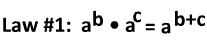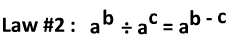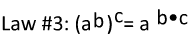Let's start by reviewing the rules for exponents

MULTIPLYING

When you multiply same bases, you add exponents.

x4•x5=x4+5=x9

What if an exponent is negative?

The same thing, add the exponents.

x6•x-4=x6+(-4) = x2
What if there is more than one variable?

Do each base separately.

(xy6)(x3y4)=x1+3y6+4=x4y10
What if there is a coefficient in front of the variable?

3x2•-2x3= (3 •-2) • (x2 • x3) = -6x5.

Use the commutative property to rearrange

-6x5   multiply the coefficients and add exponents

DIVIDING

When you divide same bases, you subtract exponents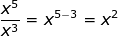What if there is more than one variable? Do each base separately.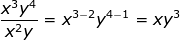What if there is a coefficient in front of the variable? Divide the coefficients.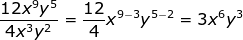What, if the exponent is negative?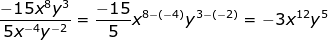### RAISING A POWER TO A POWER

When you put a power to a power you multiply exponents.

(x3)5=x3•5=x15

What if there is more than one variable?

(x2y)3=x2•3y1•3=x6y3

What if there is a coefficient?

(2x4y2)4=24x4•4y2•4=16x16y8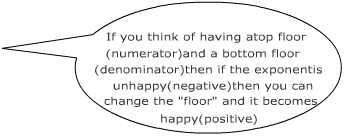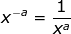### NEGATIVE EXPONENT RULEinitially write a "top floor" and "bottom floor"

2nd change floors if the exponent is "unhappy"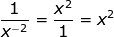The exponent is unhappy in the denominator so move to the numerator and it becomes positive. Let's look at some more challenging examples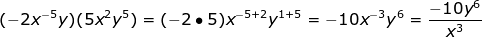Remember to work slowly and meticulously. You will need to memorize the rules for exponents. A shortened version:

• Multiply → Add exponents

• Divide → Subtract exponents

• Power to a power → Multiply exponents

• Negative → Change "floors"

## ZERO EXPONENT

The exponent of a number says how many numbers of times to use the number in a multiplication. However, a zero exponent produces a bit of a puzzle because you cannot multiply a number zero times. However, we can develop the rule from the exponent rules for the division. Any number divided by itself = 1. For example, when we look at the rules for the division which says that when you divide you subtract exponents. We get the following:   and since we already know that it follows that x° = 1. This means that any number except zero (0) raised to the zero power is 1.

These laws or short cuts can help to solve exponent questions easier. {\displaystyle b^{p^{q}}=b^{\left(p^{q}\right)},}

{\displaystyle \left(b^{p}\right)^{q}=b^{pq}.}

### Powers of ten

In the base ten number system, integer powers of 10 are written as the digit 1 preceded by several zeroes resolved by the sign and magnitude of the exponent. For example, 104= 10000 and 10−4 = 0.0001.

Exponentiation with base 10 is used in scientific notations denote large or small numbers. For instance, 299792458 m/s (the speed of light in vacuum, in meters per second) can be written as 2.99792458×108 m/s and then approximated as 2.998×108 m/s. SI Prefixes based on powers of 10 are also used to explain small or large quantities. For example, the prefix kilo means 103 = 1000, so 1000m in 1killometre.

### Powers of two

The first negative powers of 2 are used commonly and have special names, e.g.: half and quarter

Powers of 2 present in set theory, since a set with n members, has a power set, the set of all of its subsets, which has 2n members. Integer powers of 2 are important in the computer world. The positive integer powers 2n give the number of possible values for an n-bit integer binary number; for example, a byte may take 28 = 256 different values. The binary number system expresses any number as a sum of powers of 2, and denotes it as a sequence of 0 and 1, separated by a binary point. Where, 1 indicates a power of 2 that appears in the sum; the exponent is determined by the place of this 1: the nonnegative exponents are the rank of the 1 on the left of the point (starting from 0), and the negative exponents are determined by the rank on the right of the point.

### Powers of one

The powers of one are all one: 1n = 1.

### Powers of zero

If the exponent n is positive (n > 0), the nth power of zero is zero: 0n = 0.

If the exponent n is negative (n < 0), the nth power of zero 0n is undefined, because it must equal with -n > 0. {\displaystyle 1/0}

The expression 00 is either called as 1, or it is left undefined.

### Powers of negative one

If n is an even integer, then (−1)n is equal to the 1.

If n is an odd integer, then (−1)n is equal to the 1 with a negative sign.

Rational (Fractional) Exponent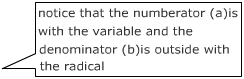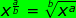Now let's practice with a few numerical expressions.

For example:

••An easy way to remember the rule is to choose an example that you know for sure. For example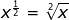. This way you can memorize that the denominator is the number that goes with the radical and the numerator goes with the number/variable under the radical.

Reminder: the exponent rules do not change when you multiply, divide or raise a power to a power because the exponent is a fraction.

••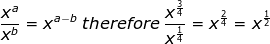•## Topics & Subtopics of NCERT Class 9 Maths Chapter 1 – Number System

The different topics that are covered in this chapter are –

• The number line and representing the real numbers on the number line.
• A rational number can be defined as a number, which can be written in the form of p/q, where p and q are integers and q is not equal to 0.
• An irrational number is a number, which cannot be written in the form of p/q, where p and q are integers and q is not equal to 0.
• Real numbers and the decimal expansions.
• Operations of real numbers.
• Laws of exponents for real numbers. The different rules surrounding the real numbers.

## Significance of NCERT Book Class 9

1. With the NCERT book, students will understand the concepts and will also learn to utilize them in the competitive exams.
2. The NCERT book provides the students with knowledge about different methods to solve a single problem. The students should focus on learning these different methods and the solved examples will help in offering a better understanding, while the unsolved questions will help in utilizing the gained knowledge effectively.
3. The book also provides the students with valuable content that explains everything in a crisp and concise manner. With this approach, students gain immense knowledge, which is extremely helpful in writing the board exams with better knowledge, as it helps in building the knowledge of the students perfectly.

In addition, with the help of Doubtnut online platform for education material, students can always clear all their doubts and confusions as the website covers all the information regarding the NCERT book Class 9 in a format that is easily understood by students. Our website provides video-tutorials and a pdf format of the book that helps students to study their course material in an interesting way.

Share this on :-